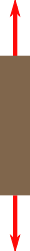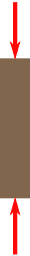# Compressive strength

﻿
Compressive strength

Compressive strength is the capacity of a material or structure to withstand axially directed pushing forces. When the limit of compressive strength is reached, materials are crushed. Concrete can be made to have high compressive strength, e.g. many concrete structures have compressive strengths in excess of 50 MPa, whereas a material such as soft sandstone may have a compressive strength as low as 5 or 10 MPa.Measuring the compressive strength of a steel drum

Compressive strength is often measured on a universal testing machine; these range from very small table top systems to ones with over 53 MN capacity. Measurements of compressive strength are affected by the specific test method and conditions of measurement. Compressive strengths are usually reported in relationship to a specific technical standard that may, or may not, relate to end-use performance.

Compare tensile strength.

## IntroductionTensionCompression

When a specimen of material is loaded in such a way that it extends it is said to be in tension. On the other hand if the material compresses and shortens it is said to be in compression.

On an atomic level, the molecules or atoms are forced apart when in tension whereas in compression they are forced together. Since atoms in solids always try to find an equilibrium position, and distance between other atoms, forces arise throughout the entire material which oppose both tension or compression.

The phenomena prevailing on an atomic level are therefore similar. On a macroscopic scale, these aspects are also reflected in the fact that the properties of most common materials in tension and compression are quite similar.[citation needed]

The major difference between the two types of loading is the strain which would have opposite signs for tension (positive—it gets longer) and compression (negative—it gets shorter).

Another major difference is tension tends to pull small sideways deflections back into alignment, while compression tends to amplify such deflection into buckling.

## Compressive Strength

By definition, the compressive strength of a material is that value of uniaxial compressive stress reached when the material fails completely. The compressive strength is usually obtained experimentally by means of a compressive test. The apparatus used for this experiment is the same as that used in a tensile test. However, rather than applying a uniaxial tensile load, a uniaxial compressive load is applied. As can be imagined, the specimen (usually cylindrical) is shortened as well as spread laterally. A Stress–strain curve is plotted by the instrument and would look similar to the following:Engineering Stress-Strain curve for a typical specimen

The compressive strength of the material would correspond to the stress at the red point shown on the curve. Even in a compression test, there is a linear region where the material follows Hooke's Law. Hence for this region$\sigma=E\epsilon$ where this time E refers to the Young's Modulus for compression.

This linear region terminates at what is known as the yield point. Above this point the material behaves plastically and will not return to its original length once the load is removed.

There is a difference between the engineering stress and the true stress. By its basic definition the uniaxial stress is given by:$\sigma = \frac{F}{A}$

where, F = Load applied [N], A = Area [m2]

As stated, the area of the specimen varies on compression. In reality therefore the area is some function of the applied load i.e. A = f(F). Indeed, stress is defined as the force divided by the area at the start of the experiment. This is known as the engineering stress and is defined by,$\sigma_e = \frac{F}{A_0}$

A0=Original specimen area [m2]

Correspondingly, the engineering strain would be defined by:$\epsilon_e = \frac{l-l_0}{l_0}$

where l = current specimen length [m] and l0 = original specimen length [m]

The compressive stress would therefore correspond to the point on the engineering stress strain curve$(\epsilon_e^*,\sigma_e^*)$ defined by$\sigma_e^* = \frac{F^*}{A_0}$$\epsilon_e^* = \frac{l^*-l_0}{l_0}$

where F* = load applied just before crushing and l* = specimen length just before crushing.

## Deviation of engineering stress from true stressBarrelling

In engineering design practice we mostly rely on the engineering stress. In reality, the true stress is different from the engineering stress. Hence calculating the compressive strength of a material from the given equations will not yield an accurate result. This is of course because the cross sectional area A0 changes and is some function of load A = φ(F).

The difference in values may therefore be summarized as follows:

• On compression, the specimen will shorten. The material will tend to spread in the lateral direction and hence increase the cross sectional area.
• In a compression test the specimen is clamped at the edges. For this reason, a frictional force arises which will oppose the lateral spread. This means that work has to be done to oppose this frictional force hence increasing the energy consumed during the process. This results in a slightly inaccurate value of stress which is obtained from the experiment.

As a final note, it should be mentioned that the frictional force mentioned in the second point is not constant for the entire cross section of the specimen. It varies from a minimum at the centre to a maximum at the edges. Due to this a phenomenon known as barrelling occurs where the specimen attains a barrel shape.

## Comparison of compressive and tensile strengths

An example of a material with a much higher compressive strength than tensile strength is concrete. Ceramics typically have a much higher compressive strength than tensile strength. Composite materials tend to have higher tensile strengths than compressive strengths. One such example is glass fiber epoxy matrix composite.

Wikimedia Foundation. 2010.

### Look at other dictionaries:

• Compressive strength — Compressive strength. См. Предел прочности при сжатии. (Источник: «Металлы и сплавы. Справочник.» Под редакцией Ю.П. Солнцева; НПО Профессионал , НПО Мир и семья ; Санкт Петербург, 2003 г.) …   Словарь металлургических терминов

• compressive strength — gniuždomasis stipris statusas T sritis Standartizacija ir metrologija apibrėžtis Didžiausias gniuždymo įtempis, kuriam esant medžiaga suyra. atitikmenys: angl. compression strength; compressive strength vok. Druckfestigkeit, f rus. прочность на… …   Penkiakalbis aiškinamasis metrologijos terminų žodynas

• compressive strength — gniuždymo stipris statusas T sritis fizika atitikmenys: angl. compression strength; compressive strength vok. Druckfestigkeit, f rus. прочность при сжатии, f pranc. résistance à la compression, f; résistance par compression, f …   Fizikos terminų žodynas

• compressive strength — noun : the maximum compressive stress that under gradually applied load a given solid material will sustain without fracture compare tensile strength …   Useful english dictionary

• compressive strength — noun the resistance of a material to breaking under compression. Compare with tensile strength …   English new terms dictionary

• compressive strength — noun The property of a material that relates to its ability to resist compression loads …   Wiktionary

• compressive strength test —       mechanical test measuring the maximum amount of compressive load a material can bear before fracturing. The test piece, usually in the form of a cube, prism, or cylinder, is compressed between the platens of a compression testing machine by …   Universalium

• Compressive stress — is the stress applied to materials resulting in their compaction (decrease of volume). When a material is subjected to compressive stress, then this material is under compression. Usually, compressive stress applied to bars, columns, etc. leads… …   Wikipedia

• Strength — is the amount of force that a muscle or group of muscles can exert.Strength may refer to:Physical ability: *Physical strength, as in people or animals *Superhuman strength, as in fictional characters *a character attribute (role playing… …   Wikipedia

• Strength of materials — Internal force lines are denser near the hole, a common stress concentration In materials science, the strength of a material is its ability to withstand an applied stress without failure. The applied stress may be tensile, compressive, or shear …   Wikipedia

We are using cookies for the best presentation of our site. Continuing to use this site, you agree with this.